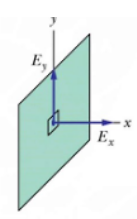Courses
Courses for Kids
Free study material
Offline Centres
MoreLast updated date: 09th Dec 2023
Total views: 280.8k
Views today: 2.80k

# An infinite large plate has a net positive charge. When referring to the electric field just as it is coming out of the plate:A. Some of the electric field is perpendicular to the plateB. All of the electric field is perpendicular to the plateC. None of the electric field is perpendicular to the plateD. There is no electric field coming out of the plateVerified
280.8k+ views
Hint: The electric force per unit charge is known as the electric field. The force that the field will apply on a positive test charge is assumed to be in the same direction as the field's direction. A positive charge's electric field is radially outward, and a negative charge's field is radially inward.

Even if the rule by itself is insufficient to evaluate the electric field over a surface enclosing some charge distribution, in situations where symmetry requires uniformity of the field, this could be possible. The cumulative flux associated with a closed surface is $\dfrac{1}{{{\varepsilon _o}}}$ times the charge enclosed by the closed surface, according to the Gauss theorem.
According to Gauss's theorem, all electric field lines originating from an infinitely charged plate are perpendicular to the plate.Magnitude of electric field ${\rm{E}} = \dfrac{\sigma }{{2{_{\rm{o}}}}}$.